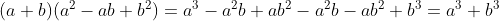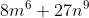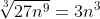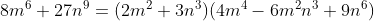## Sum of Two Cubes

A binomial of the form of the sum of two cubed terms can be factored in the formThis identity can be easily proven by multiplying the factors to the right side:This can be applied to more complex sums of cubes:We take the cube root of the first termAnd the cube root of the second termThen the binomial is factored as